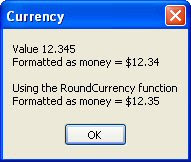## Sunday, June 10, 2012

### Rounding the Currency type like they taught us in school

I started using Delphi's Currency type for it's accuracy only to discover it doesn't round the way I would have expected it to round. I remember being taught in school that when rounding numbers if the remainder was equal to or greater than five you round up otherwise you round down.

For Example:
• 12.344 Rounds to 12.34
• 12.345 Rounds to 12.35
• 12.346 Rounds to 12.35
This is how I was taught in school.
This is how Microsoft Excel does it when you set the cell to display as two decimal places.
This is how Microsoft SQL does it when you format the display as two decimal places.

```'---------------------------------
' Microsoft SQL
'---------------------------------
DECLARE @Money money
SET @Money = 12.345
SELECT CONVERT (varchar(10),@Money,1)
'---------------------------------
12.35
```
But that's not how Delphi does it.

```'---------------------------------
' Delphi 2010
'---------------------------------
procedure TForm1.Button1Click(Sender: TObject);
var
s : string;
c : currency;
begin
c := 12.345;
s := '';
s := s + 'Value ' + FloatToStr(c);
s := s + Chr(13);
s := s + Format('Formatted as money = %m',[c]);
ShowMessage(s);
end;
```So this lead me looking around for an answer. It appears that Delphi uses what is known as Bankers Rounding which means round to the closest even number. I was never taught this in school. This is how Bankers Rounding works:
• 12.345 Rounds to 12.34
• 12.355 Rounds to 12.36
• 12.365 Rounds to 12.36
I do not want this type of rounding behavior... I want the rounding that I was taught in school. The problem is Delphi's internals do not support this type of rounding. (This is where I have one of those love hate realtionships with Delphi. I hate Delphi!)

So I posed a question on StackOverflow and after clarifying what I meant, got a couple interesting responses. Here is a solution I found reading through the Embarcadero discussion mentioned in my question on SO.
```function RoundCurrency(const Value: Currency): Currency;
var
V64: Int64 absolute Result;
Decimals: Integer;
begin
Result := Value;
Decimals := V64 mod 100;
Dec(V64, Decimals);
case Decimals of
-99 .. -50 : Dec(V64, 100);
50 .. 99 : Inc(V64, 100);
end;
end;

procedure TForm1.Button1Click(Sender: TObject);
var
s : string;
c : currency;
begin
c := 12.345;
s := '';
s := s + 'Value ' + FloatToStr(c);
s := s + Chr(13);
s := s + Format('Formatted as money = %m',[c]);
s := s + Chr(13);
s := s + Chr(13);
s := s + 'Using the RoundCurrency function';
s := s + Chr(13);
s := s + Format('Formatted as money = %m',[RoundCurrency(c)]);
ShowMessage(s);
end;
```Now that's more like it. I love Delphi!

Enjoy
Semper Fi - Gunny Mike